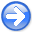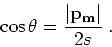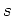Time-shift imaging condition in seismic migrationNext: Moveout analysis Up: Angle transformation in wave-equation Previous: Space-shift imaging condition

## Time-shift imaging condition

Using the same definitions as the ones introduced in the preceding subsection, we can re-write equation (18) as(22)

from which we can derive an expression for angle-transformation after time-shift prestack imaging:(23)

Relation (23) can be interpreted using ray parameter vectors at image locations (Figure 2).img3
Figure 2.
Interpretation of angle-decomposition based on equation (23) for time-shift gathers.Angle-domain common-image gathers can be obtained by transforming prestack migrated images using equation (23):(24)

Equation (23) can be written as(25)

whereare partial derivatives ofrelative to. We can rewrite equation (25) as(26)

wheredenote partial derivative of coordinaterelative to coordinatesand, respectively. Equation (26) describes an algorithm in two steps for angle-decomposition after time-shift imaging: computethrough a slant-stack inpanels (find a change inwith respect to), then apply a correction using the migration slownessand a function of the structural dips.Time-shift imaging condition in seismic migrationNext: Moveout analysis Up: Angle transformation in wave-equation Previous: Space-shift imaging condition

2007-04-08